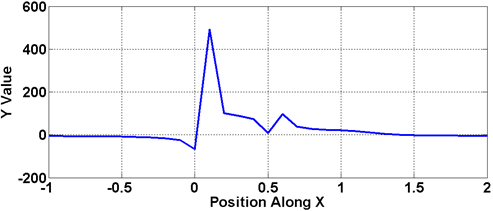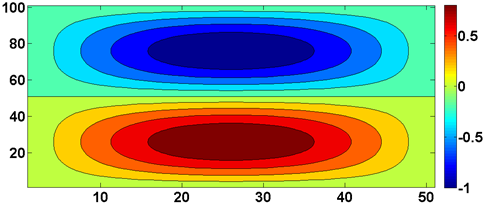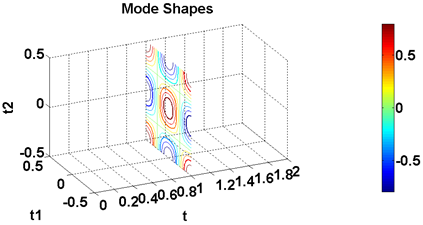# MATLAB Resources

Introduction to MATLAB and MATLAB Scripting

## Plotting using Matlab

• Plotting is done to visualize the data
• Plotting can be done from measured data or from an equation or from simulation
• Types of Plotting are

a) XY Plot

b) 2- dimensional plot

c) 3 - dimensional plot

## Plotting Examples

• Line plots are used to represent a function of one variable

%%%%%%%%
%
%Illustration to plot
%
%%%%%%%%

X=-1:0.1:2;

Y=humpchanged(X);         %User defined Function

plot(X,Y);## Two dimensional Plots

• Two dimensional plots are used to represent a function of two variables%%%%%%%%%%%
%
%
%Illustration to Plot
%Images together
%
%%%%%%%%%%%

t=0:0.01:1;

t1=-0.25:0.01:0.25;

y=sin(2*pi*t);

y1=cos(2*pi*t1);

kk=y.'*y1;

figure(2)

contourf(kk);

colorbar

## Three Dimensional Plots

• Three dimensional plots are used to represent a function of three variables%%%%%%%%%%%%%%%%%%%%%%
%
%Illustration to plot
%variation of many
%variables
%
%%%%%%%%%%%%%%%%%%%%%%%

clear all
clc

values=zeros(101,101,101);

t=0:0.01:1;

t1=-0.5:0.01:0.5;

t2=-0.5:0.01:0.5;

for kk=1:1:101
for jj=1:1:101
for ii=1:1:101
values(ii,jj,kk)=cos(2*pi*t(ii))*cos(2*pi*t1(jj))*cos(2*pi*t2(kk));
end
end
end

figure
colormap jet
contourslice(t,t1,t2,values,1:1:51,1:1:101,1:1:101)
xlabel('t')
ylabel('t1')
zlabel('t2')
colorbar
view(-11,14)
%axis equal

University of Cincinnati Libraries

PO Box 210033 Cincinnati, Ohio 45221-0033

Phone: 513-556-1424

University of Cincinnati